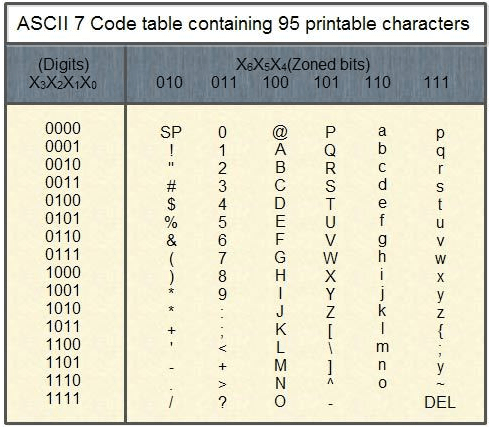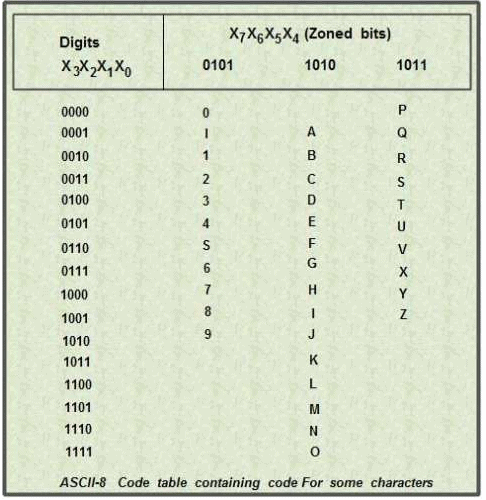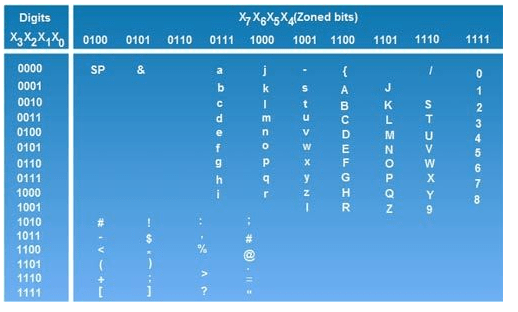# Alphanumeric Code Electrical Engineering (EE) Notes | EduRev

## Electronics and Communication Engineering (ECE) : Alphanumeric Code Electrical Engineering (EE) Notes | EduRev

The document Alphanumeric Code Electrical Engineering (EE) Notes | EduRev is a part of the Electronics and Communication Engineering (ECE) Course Digital Electronics.
All you need of Electronics and Communication Engineering (ECE) at this link: Electronics and Communication Engineering (ECE)

## Alphanumeric Codes

Earlier computers were used only for the purpose of calculations i.e. they were only used as a calculating device. But now computers are not just used for numeric representations, they are also used to represent Information such as names, addresses, item descriptions etc. Such information is represented using letters and symbols.  computer is a digital system and can only deal with l's and 0’s. So to deal with letters and symbols they use alphanumeric codes.

Alphanumeric codes, also called character codes, are binary codes used to represent alphanumeric data. The codes write alphanumeric data, including letters of the alphabet, numbers, mathematical symbols and punctuation marks, in a form that is understandable and process able by a computer. Using these codes, we can interface input-output devices such as keyboards, monitors, printers etc. with computer.

Several coding techniques have been invented that represent alphanumeric information as a series of l's and O's. The earliest better-known alphanumeric codes were the Morse code used in telegraph and 12-bit Hollerith code used when punch cards were used as a medium of inputting and outputting data. As the punched cards have completely vanished with the evolution of new mediums, the Hollerith code was rendered obsolete. Now the ASCII and EBCDIC codes are the two most widely used alphanumeric codes. The ASCII Code is very popular code used in all personal computers and workstations whereas the EBCDIC code is mainly alphanumeric code, the UNICODE, has evolved to overcome the limitation of limited character encoding as in case of ASCII and EBCDIC code.

## Types of Alphanumeric Code.

### MORSE CODE

The Morse code, invented in 1837 by Samuel F.B. Morse, was the first alphanumeric code used in telecommunication. It uses a standardized sequence of short and long elements to represent letters, numerals and special characters of a given message. The short and long elements can be formed by sounds, marks, pulses, on off keying and are commonly known as dots and dashes. For example : The letter 'A' is formed by a dot followed by a dash. The digit 5 is formed by 5 dots in succession. The International Morse code treats a dash equal to three dots. To see the details of Morse code table you can refer the Internet search engines.

Due to variable length of Morse code characters, the morse code could not adapt to automated circuits. In most electronic communication, the Baudot code and ASCII code are used.

Morse code has limited applications. It is used in communication using telegraph lines, radio circuits. Pilots and air traffic controllers also use them to transmit their identity and other information.

### BAUDOT CODE

The Baudot code was another popular alphanumeric code used in early 1860's. It was invented by the French engineer Emile Baudot in 1870. It is a 5-unit code (i.e. it uses five elements to represent an alphabet). Moreover, unlike the Morse code, all the symbols all of equal duration. This allowed telegraph transmission of the Roman alphabet and punctuation and control signals.

### HOLLERITH CODE

In 1896, Herman Hollerith formed a company called the Tabulating Machine Company. This company developed a line of machines that used punched cards for tabulation. After a number of mergers, this company was formed into the IBM, Inc. We often refer to the punched-cards used in computer systems as Hollerith cards and the 12-bit code used on a punched-card is called the Hollerith code.

A Hollerith string is a sequence of l2-bit characters; they are encoded as two ASCII characters, containing 6 bits each. The first character contains punches 12,0,2,4,6,8 and the second character contains punches 11, 1,3,5, 7, 9. Interleaving the two characters gives the original 12 bits. To make the characters printable on ASCII terminals, bit 7 is always set to 0 and bit 6 is said to the complement of bit 5. These two bits are ignored when reading Hollerith cards.

Today, as punched cards are mostly obsolete and replaced with other storage medias so the Hollerith code is rendered obsolete.

American Standard-Code for information Interchange (ASCII)

The American Standard-Code for Information Interchange (ASCII) pronounced "as-kee" is a 7-bit code based on the ordering of the English alphabets. The ASCII codes are used to represent alphanumeric data in computer input/output.

Historically, ASCII developed from telegraphic codes. It was first published as a standard in 1967. It was subsequently updated and many versions of it were launched with the most recent update in 1986. Since it is a seven-bit code, it can almost represent 128 characters. These include 95 printable characters including 26 upper-case letters (A to Z), 26 lowercase letters (a to z), 10 numerals (0 to 9) and 33 special characters such as mathematical symbols, space character etc. It also defines codes for 33 non-printing obsolete characters except for carriage return and/or line feed. The below table lists the 7 bit ASCII code containing the 95 printable characters.The format of ASCII code for each character is X6·Xs, X4, X3, X2, Xl' X0 where each X is 0’ or 1. For instance, letter D is coded as .1000100. For making reading easier, we leave space as follows: 1000100.

Similarly, from the above table, we see that letter 'A' has X6 X5X4 of 100 and X3 X2 XXo of 0001 (A). Similarly, the digit '9' has X6 X5 X4 values of 011 and X3 X2 XX0 of 1001 so the ASCII-7 code for digit 9 is 0111001.

More examples are:

The ASCll-7 code for 'd' is 1100100 as seen from the table 3.4.

The ASCll-7 code for '+' is 0101011 as seen from the table 3.4.

An eight-bit version of the ASCII code, known as US ASCII-8 or ASCII-8, has also been developed. Since it uses 8-bits, so this version of ASCII can represent a maximum of 256 characters.

The table below lists some ASCII-8 codes.

When the ASCII -7 codes was introduced, many computers dealt with eight -bit groups (or bytes) as the smallest unit of information. This eight bit code was commonly used as parity bit for detection of error on communication lines. Machines that did not use the parity bit typically set the eighth bit to O. In that case, the ASCII code format would be X7 X6 X5 X4 X3 X2 Xl X0' In case of ASCII-7 code, if this representation is chosen to represent characters then X7 would always be zero. So, the eight-bit ASCII-7 code for 'A’ would be 01000001 and for 4' would be 00101011.Example 1: With an ASCII-7 keyboard, each keystroke produces the ASCII equivalent of the designated character. Suppose that you type PRINT X. What is the output of an ASCII-7 keyboard?

Solution: The sequence is as follows:

The ASCII-7 equivalent of P = 101 0000

The ASCII-7 equivalent of R = 101 0010

The ASCII-7 equivalent of I = 1001010

The ASCII-7 equivalent of N = 100 1110

The ASCII-7 equivalent of T = 1-010100

The ASCII-7 equivalent of space = 010 0000

The ASCII-7 equivalent of X = 101 1000

So the output produced is 1010000101001010010101001110101010001000001011000. The output in hexadecimal equivalent is 50 52 49 4E 54 30 58

Example2: A computer sends a message to another computer using an odd-parity bit. Here is the message in ASCII-8 Code.

1011 0001

1011 0101

1010 0101

1010 0101

1010 1110

What do these numbers mean?

Solution

On translating, the 8-bit numbers into their equivalent ASCII-8 code we get the word 1011 0001 (Q), 10110101 (U), 10100101 (E), 1010 0101 (E), 1010 1110 (N)

So, on translation we get QUEEN as the output.

### Extended Binary Coded Decimal Interchange Code (EBCDIC)

The Extended Binary Coded Decimal Interchange Code (EBCDIC) pronounced as "ebi-si disk" is another frequently used code by computers for transferring alphanumeric data. It is 8-bit code in which the numerals (0-9) are represented by the 8421 BCD code preceded by 1111. Since it is a 8-bit code, it can almost represent 23 (= 256) different characters which include both lowercase and uppercase letters in addition to various other symbols and commands.EBCDIC was designed by IBM corp. so it is basically used by several IBM· models. In this code, we do not use a straight binary sequence for representing characters, as was in the case of ASCII code. Since it is a 8-bit code, so it can be easily grouped into groups of 4 so as to represent in arm of hexadecimal digits. By using the hexadecimal number system notation, the amount of digits used to represent various characters and special characters using EBCDIC code is reduced in volume of one is to four. Thus 8-bit binary code could be reduced to 2 hexadecimal digits which are easier to decode if we want to view the internal representation in memory. The above table lists the EBCDIC code for certain characters.

Read the above table as you read the graph. Suppose you want to search for EBCDIC code for letter 'A’. To that case, the value of X3 X2 Xl X0 bits is 0001 and value X7 X6 XX4 bits is 1100.

Therefore, EBCDIC code for letter 'A’ is 11000001(A). Similarly, the EBCDIC code for 'B' is 11000010(B).

The EBCDIC code '=' is 01111110

The EBCDIC code for '\$' is 0101 1011

### UNICODE

The ASCII and EBCDIC encodings and their variants that we have studied suffer from some limitations.

1. These encodings do not have a sufficient number of characters to be able to encode alphanumeric data of all forms, scripts and languages. As a result, they do not permit multilingual computer processing.

2. These encoding suffer from incompatibility. For example: code 7A (in hex) represents the lowercase letter 'Z' in ASCII code and the semicolon sign ';' in EBCDIC code.

To overcome these limitations, UNICODE also known as universal code was developed jointly by the Unicode Consortium and the International Organization for Standardization (ISO). The Unicode is a 16-bit code so it can represent 65536 different characters. It is the most complete character encoding scheme that allows text of all forms and languages to be encoded for use by computers. In addition to multilingual support, it also supports a comprehensive set of mathematical and technical symbols, greatly simplifying any scientific information interchange.

UNICODE has a number of uses

1. It is increasingly being used for internal processing and storage of text. Window NT and its descendents, Java environment, Mac OS all follow Unicode as the sole internal character encoding.

2. All World Wide Web consortium recommendations have used Unicode as their document character set since HTML 4.0.

3. It partially addresses the new line problem that occurs when trying to read a text file on different platforms. It defines a large number of characters that can be recognized as line terminators.

Unicode is currently being adopted by top computer industry leaders like Microsoft, Apple, Oracle, Sun, SAP and many more in their products.

Offer running on EduRev: Apply code STAYHOME200 to get INR 200 off on our premium plan EduRev Infinity!

## Digital Electronics

91 videos|75 docs|8 tests

,

,

,

,

,

,

,

,

,

,

,

,

,

,

,

,

,

,

,

,

,

;